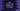# How to find random numbers in a range in Dart## Introduction :

Write one dart program to find random numbers in a range. Dart math library dart:math provides one function to create random numbers. We will use this function to find random numbers in a range.

### Dart math random :

Dart nextInt method is used to create random numbers. It is defined as below :

``int nextInt(int maxValue)``

It is defined in dart:math library. So, we need to import dart:math to use this method. It generates one non-negative random integer in the range of 0(inclusive) to maxValue(exclusive).

### Dart math random example :

``````import 'dart:math';
main(List<string> args) {
final random = new Random();
for (int i = 0; i < 10; i++) {
print(random.nextInt(100));
}
}``````

This program will print 10 random numbers between 0 and 100.

Each time you execute this program, it will print different numbers.

### Dart program to find random numbers in range :

We don’t have any other function to find random numbers between two numbers. random method returns one random value between 0 and the argument value we pass to this method. So, to find random numbers between two numbers say min and max, we can pass max - min as the parameter to this method and add min to that result.

This will always give one random value between min and max.

``````import 'dart:math';
main(List<string> args) {
final random = new Random();
final min = 100;
final max = 500;
for (int i = 0; i < 10; i++) {
print(min + random.nextInt(max - min));
}
}``````

This program will print ten random numbers between 100 and 500.